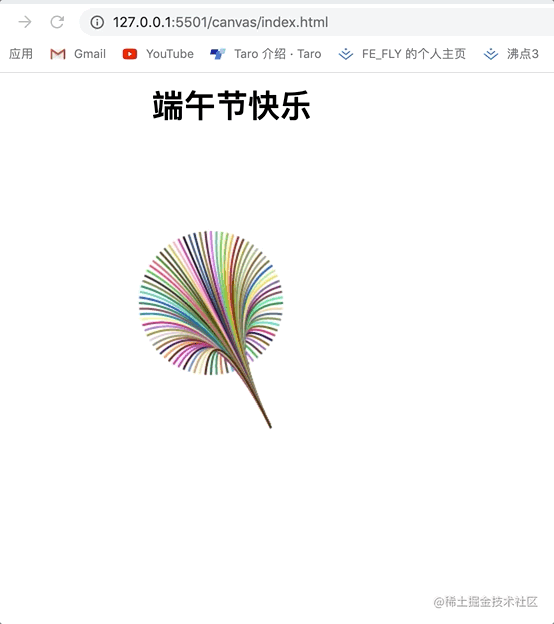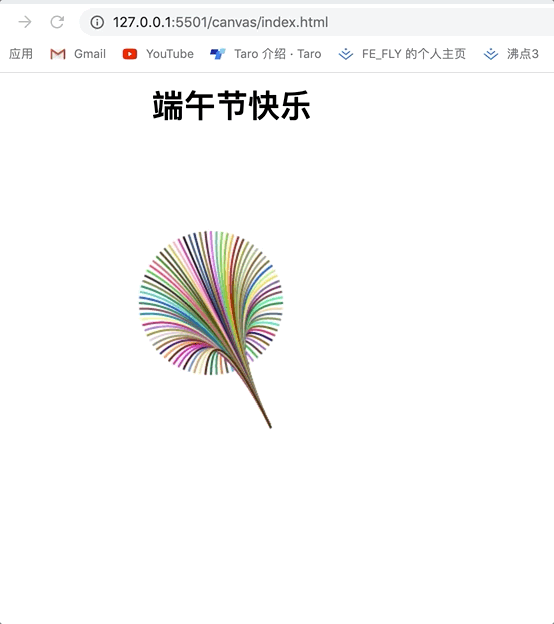# 面试官问我会canvas? 我可以绘制一个烟花🎇动画

## 前言

1. svg 中的曲线（支持 2阶、 3阶）
2. canvas 中绘制贝塞尔曲线
3. 几乎所有前端2D或3D图形图表库(echarts，d3，three.js)都会使用到贝塞尔曲线

# 绘制贝塞尔曲线

``````const canvas = document.getElementById( 'canvas' );
const ctx = canvas.getContext( '2d' );
ctx.beginPath();
ctx.lineWidth = 2;
ctx.strokeStyle = '#000';
ctx.moveTo(100,100)
ctx.stroke();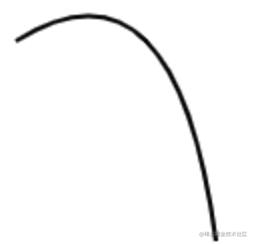# 绘制贝塞尔曲线动画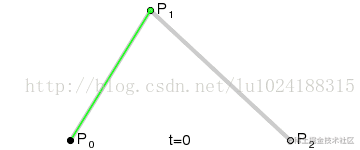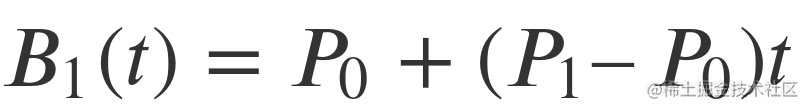``````//这个就是二阶贝塞尔曲线方程
function twoBezizer(p0, p1, p2, t) {
const k = 1 - t
return k * k * p0 + 2 * (1 - t) * t * p1 + t * t * p2
}

//离散
function drawWithDiscrete(ctx, start, control, end,percent) {
for ( let t = 0; t <= percent / 100; t += 0.01 ) {
const x = twoBezizer(start, control, end, t)
const y = twoBezizer(start, control, end, t)
ctx.lineTo(x, y)
}
}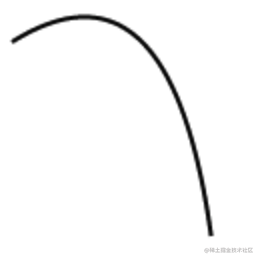``````let percent = 0
function animate() {
ctx.clearRect( 0, 0, 800, 800 );
ctx.beginPath();
ctx.moveTo(100,100)
drawWithDiscrete(ctx,[100,100],[180,50],[200,200],percent)
ctx.stroke();
percent = ( percent + 1 ) % 100;
id =  requestAnimationFrame(animate)
}
animate()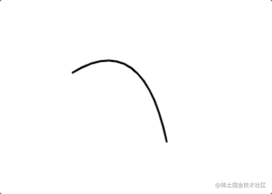# 绘制贝塞尔曲线动画方法2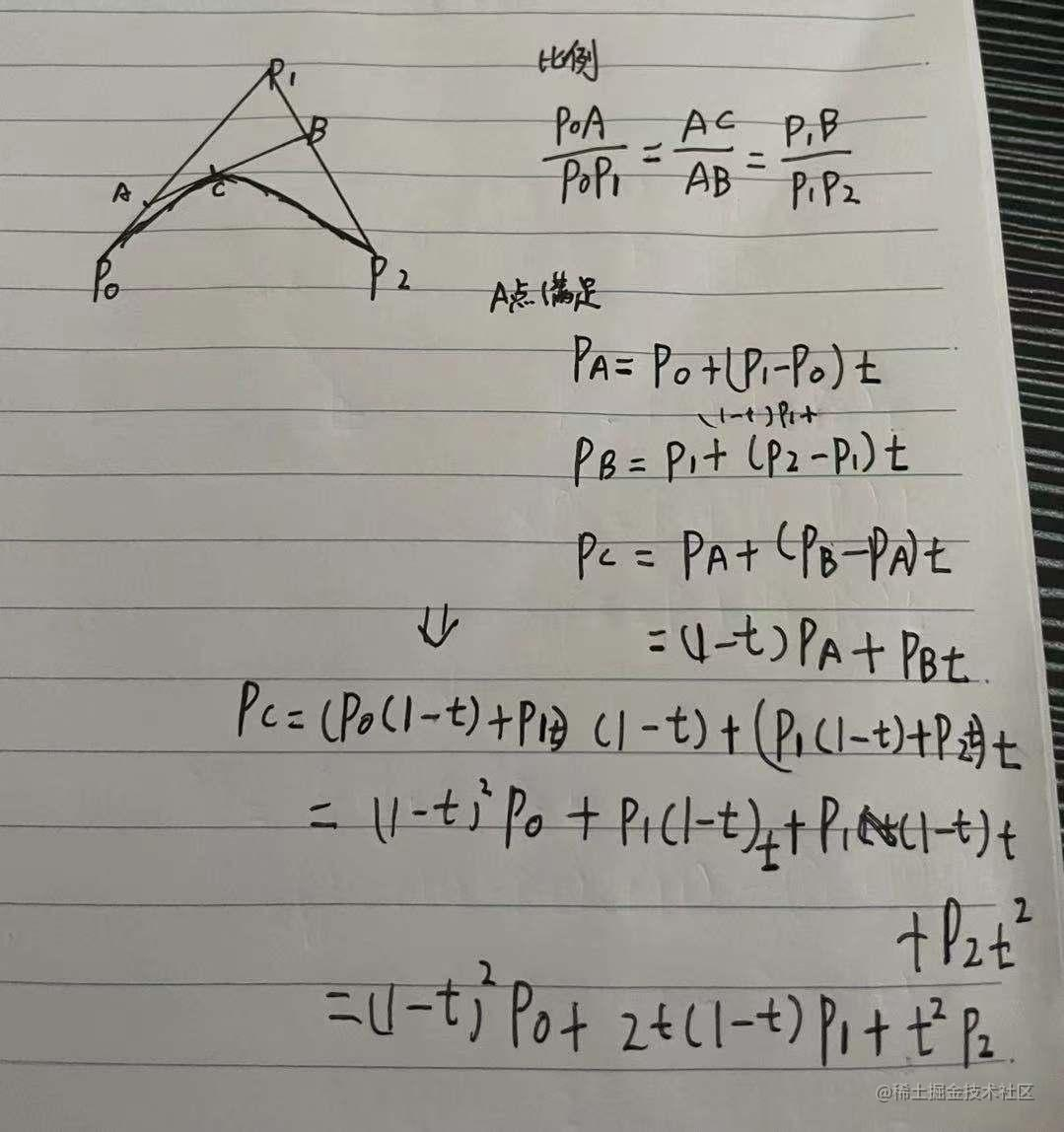`````` function oneBezizer(p0,p1,t) {
return p0 + (p1-p0) * t
}

``````function drawWithDiscrete2(ctx, start, control, end,percent) {
const t = percent/ 100;
// 求出A点
const A = [];
const C = [];
A = oneBezizer(start,control,t);
A = oneBezizer(start,control,t);
C = twoBezizer(start, control, end, t)
C = twoBezizer(start, control, end, t)
A[ 0 ], A [ 1 ],
C[ 0 ], C[ 1 ]
);
}# 礼花🎉动画

``````for(let i=0; i<count; i++) {
const angle = Math.PI * 2 / count * i;
const x = center[ 0 ] + radius * Math.sin( angle );
const y = center[ 1 ] + radius * Math.cos( angle );
ctx.strokeStyle = colors[ i ];
ctx.beginPath();
drawWithDiscrete(ctx, center,[180,50],[x,y],percent)
ctx.stroke();
}

function getRandomColor(colors, count) {
// 生成随机颜色
for ( let i = 0; i < count; i++ )  {
colors.push(
'rgb( ' +
( Math.random() * 255 >> 0 ) + ',' +
( Math.random() * 255 >> 0 ) + ',' +
( Math.random() * 255 >> 0 ) +
' )'
);
}
}